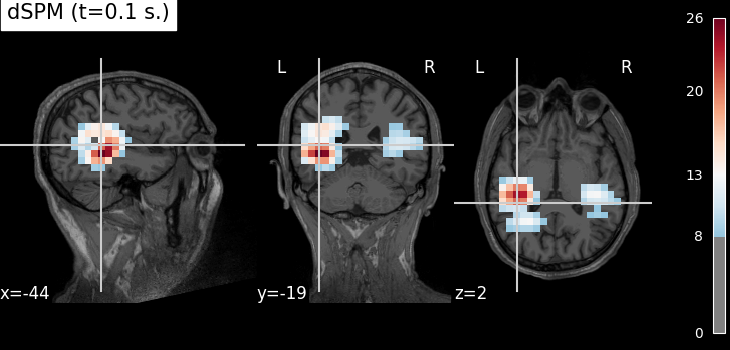# Compute MNE-dSPM inverse solution on evoked data in volume source space#

Compute dSPM inverse solution on MNE evoked dataset in a volume source space and stores the solution in a nifti file for visualisation.

```# Author: Alexandre Gramfort <alexandre.gramfort@inria.fr>
#
```
```from nilearn.image import index_img
from nilearn.plotting import plot_stat_map

from mne.datasets import sample

print(__doc__)

data_path = sample.data_path()
meg_path = data_path / "MEG" / "sample"
fname_inv = meg_path / "sample_audvis-meg-vol-7-meg-inv.fif"
fname_evoked = meg_path / "sample_audvis-ave.fif"

snr = 3.0
lambda2 = 1.0 / snr**2
method = "dSPM"  # use dSPM method (could also be MNE or sLORETA)

evoked = read_evokeds(fname_evoked, condition=0, baseline=(None, 0))
src = inverse_operator["src"]

# Compute inverse solution
stc = apply_inverse(evoked, inverse_operator, lambda2, method)
stc.crop(0.0, 0.2)

# Export result as a 4D nifti object
img = stc.as_volume(src, mri_resolution=False)  # set True for full MRI resolution

# Save it as a nifti file
# nib.save(img, 'mne_%s_inverse.nii.gz' % method)

t1_fname = data_path / "subjects" / "sample" / "mri" / "T1.mgz"
```
```Reading /home/circleci/mne_data/MNE-sample-data/MEG/sample/sample_audvis-ave.fif ...
Read a total of 4 projection items:
PCA-v1 (1 x 102) active
PCA-v2 (1 x 102) active
PCA-v3 (1 x 102) active
Average EEG reference (1 x 60) active
Found the data of interest:
t =    -199.80 ...     499.49 ms (Left Auditory)
0 CTF compensation matrices available
nave = 55 - aspect type = 100
Projections have already been applied. Setting proj attribute to True.
Applying baseline correction (mode: mean)
Reading inverse operator decomposition from /home/circleci/mne_data/MNE-sample-data/MEG/sample/sample_audvis-meg-vol-7-meg-inv.fif...
[done]
[done]
305 x 305 full covariance (kind = 1) found.
Read a total of 4 projection items:
PCA-v1 (1 x 102) active
PCA-v2 (1 x 102) active
PCA-v3 (1 x 102) active
Average EEG reference (1 x 60) active
11271 x 11271 diagonal covariance (kind = 2) found.
Did not find the desired covariance matrix (kind = 6)
11271 x 11271 diagonal covariance (kind = 5) found.
Did not find the desired covariance matrix (kind = 3)
[done]
Read a total of 4 projection items:
PCA-v1 (1 x 102) active
PCA-v2 (1 x 102) active
PCA-v3 (1 x 102) active
Average EEG reference (1 x 60) active
Source spaces transformed to the inverse solution coordinate frame
Preparing the inverse operator for use...
Scaled noise and source covariance from nave = 1 to nave = 55
Created the regularized inverter
Created an SSP operator (subspace dimension = 3)
Created the whitener using a noise covariance matrix with rank 302 (3 small eigenvalues omitted)
Computing noise-normalization factors (dSPM)...
[done]
Applying inverse operator to "Left Auditory"...
Picked 305 channels from the data
Computing inverse...
Eigenleads need to be weighted ...
Computing residual...
Explained  59.7% variance
Combining the current components...
dSPM...
[done]
```

Plot with nilearn:

```plot_stat_map(
index_img(img, 61),
str(t1_fname),
threshold=8.0,
title="%s (t=%.1f s.)" % (method, stc.times),
)
```Total running time of the script: (0 minutes 10.731 seconds)

Estimated memory usage: 501 MB

Gallery generated by Sphinx-Gallery